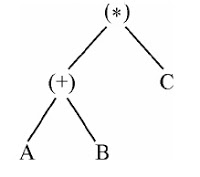41. Which of the following command the file names in multiple columns? a. IS–X b. IS c. IS–1 d. IS–f–X

 43. Which of the following expression is represented by the parse tree?a. (A + B) * C b. A + * BC c. A + B * C d. A * C + B
 Answer: (a).(A + B) * C

 44. Consider the following left associative operators in decreasing order of precedence:– subtraction (highest precedence)* multiplication$exponentiation (lowest precedence)What is the result of the following expression?3 – 2 * 4$ | * 2**3 a. – 61 b. 64 c. 512 d. 4096

 45. Which of the following is the most general phase structured grammar? a. Regular b. Context-sensitive c. Context free d. None of the above

 46. Which of the following is used for grouping of characters into tokens (in a computer)? a. A parser b. Code optimizer c. Code generator d. Scanner

 47. P: “Program is a step by step execution of the instructions”. Given P, which of the following is true? a. Program is a subset of an instruction set. b. Program is a sequence of a subset of an instruction set. c. Program is a partially ordered set of an instruction set. d. All of the above
 Answer: (b).Program is a sequence of a subset of an instruction set.

 48. In a MIU puzzle, either of the letters M, I or U could go as a start symbol. Production rules are given below :R1 : U→IUR2 : M.x→M.x.x where ːˑ is string concatenation operator. Given this, which of the following holds for(i) MIUIUIUIUIU(ii) MIUIUIUIUIUIUIUIU a. Either (i) or (ii) but not both of these are valid words. b. Both (i) and (ii) are valid words and they take identical number of transformations for the production. c. Both (i) and (ii) are valid words but they involve different number of transformations in the production. d. None of these
 Answer: (c).Both (i) and (ii) are valid words but they involve different number of transformations in the production.

 49. Recursive functions are executed in a a. First in first out-order b. Last in first out-order c. Parallel fashion d. Load balancing